Courses

# Organic CHEMISTRY - 1

## 60 Questions MCQ Test | Organic CHEMISTRY - 1

Description
This mock test of Organic CHEMISTRY - 1 for IIT JAM helps you for every IIT JAM entrance exam. This contains 60 Multiple Choice Questions for IIT JAM Organic CHEMISTRY - 1 (mcq) to study with solutions a complete question bank. The solved questions answers in this Organic CHEMISTRY - 1 quiz give you a good mix of easy questions and tough questions. IIT JAM students definitely take this Organic CHEMISTRY - 1 exercise for a better result in the exam. You can find other Organic CHEMISTRY - 1 extra questions, long questions & short questions for IIT JAM on EduRev as well by searching above.
QUESTION: 1

Solution:
QUESTION: 2

Solution:
QUESTION: 3

### The compounds A, B and C in the reaction sequence: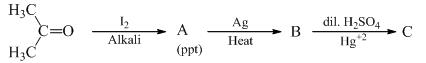Are given by the set

Solution:
QUESTION: 4

The major product formed in the following reaction is: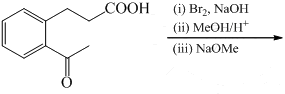Solution:
QUESTION: 5

What could be the product for the following reaction?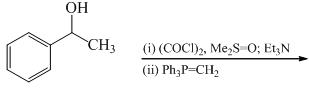Solution:
QUESTION: 6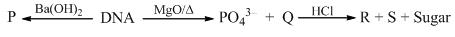In the scheme shown above P, Q, R and S are:

Solution:
QUESTION: 7

The complementary strand of DNA for the following single stranded DNA sequence: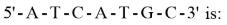Solution:
QUESTION: 8

The major product formed in the following reaction is: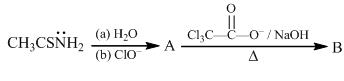Solution:
QUESTION: 9

The major product is: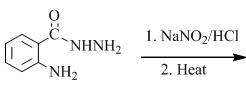Solution:
QUESTION: 10

The most suitable reagents for the following transformation is: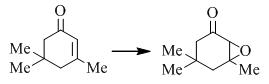Solution:
QUESTION: 11

A ketone on treatment with bromine in methanol gives the corresponding monobromo compound [P] having molecular formula C5H9BrO. The compound [P] when treated with sodium methoxide in methanol produces [Q] as the major product, the 1H NMR spectrum data for compound [P] are: 1H NMR:δ 1.17 (d, 6H), 3.02 (m, 1H), 4.10 (s, 2H). The compound P and Q , respectively is:

Solution:
QUESTION: 12

The major product formed in the following reaction is: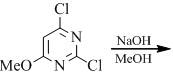Solution:
QUESTION: 13

The major product form in the following reaction is: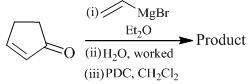Solution:
QUESTION: 14

The major product in the following reaction is: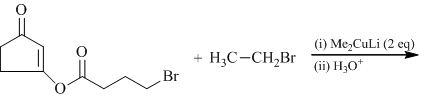Solution:
QUESTION: 15

The A and B in the following reaction sequence is: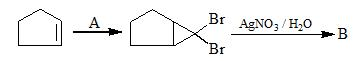Solution:
QUESTION: 16

The major product formed in the following reaction is: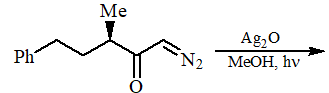Solution:
QUESTION: 17

The major product formed in the following reaction is: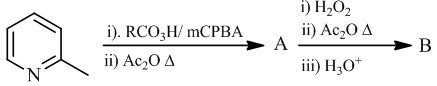Solution:
QUESTION: 18

The major product formed in the following reaction is: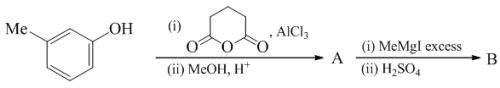Solution:
QUESTION: 19

The major product formed in the following reaction is: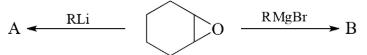Solution:
QUESTION: 20

The major product formed in the following reaction is: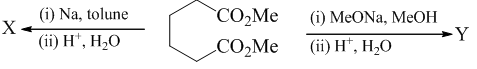Solution:
QUESTION: 21

The major product formed in the following reaction is: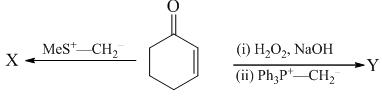Solution:
QUESTION: 22

The major product formed in the following reaction is: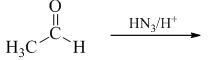Solution:
QUESTION: 23

The correct order of the rate constant for the following series of reaction (Z = CF3/CH3/OCH3) is:Solution:
QUESTION: 24

The major product formed in the following reaction is: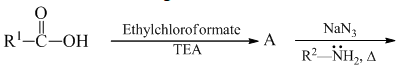Solution:
QUESTION: 25

The major product formed in the following reaction is: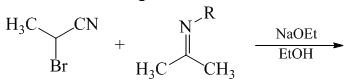Solution:
QUESTION: 26

The sequence of an mRNA molecule produced from a DNA template strand with the composition 5`-AGCTACACT-3`is:

Solution:
QUESTION: 27

In the following extraction procedure, choose the number where nicotine would be found: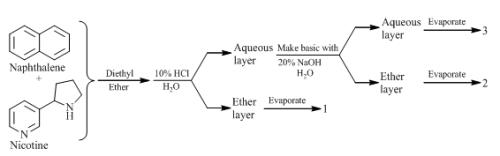Solution:
QUESTION: 28

The major product formed in the following reaction is: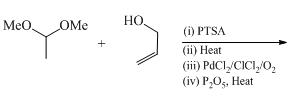Solution:
QUESTION: 29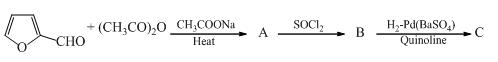Product C is:

Solution:
QUESTION: 30

Which of the following is incorrect for the final product of the given sequence of reaction: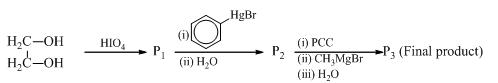Solution:
*Multiple options can be correct
QUESTION: 31

Identify correctly matched reactions with their products:

Solution:
*Multiple options can be correct
QUESTION: 32

The product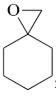is /are obtained by the following reactions:

Solution:
*Multiple options can be correct
QUESTION: 33

How many reaction is/are involve in alkyne formation:

Solution:
*Multiple options can be correct
QUESTION: 34

Identify compounds which are unstable at room temperature:

Solution:
*Multiple options can be correct
QUESTION: 35

Among the following reactions, which form salicylic acid (after acidification)?

Solution:
*Multiple options can be correct
QUESTION: 36

The reaction may gives the following possible product/s: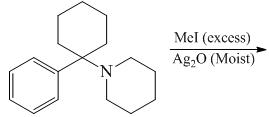Solution:
*Multiple options can be correct
QUESTION: 37

Among the following diene, how manyof compounds that undergoes a degenerate cope rearrangement reaction is/are:

Solution:
*Multiple options can be correct
QUESTION: 38

The reactions in which Cl2/NaOH is/are used as reagent:

Solution:
*Multiple options can be correct
QUESTION: 39

The reactions in which Na/Liq.NH3/EtOH is/are used as reagent:

Solution:
*Multiple options can be correct
QUESTION: 40

The reactions in which NaNH2/Liq.NH3is/are used as reagent:

Solution:
*Answer can only contain numeric values
QUESTION: 41

Number of correct statement about given reaction: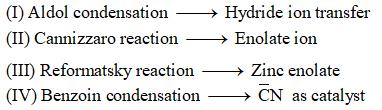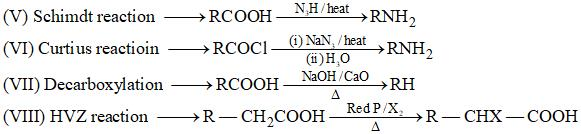Solution:
*Answer can only contain numeric values
QUESTION: 42

How many number of reaction from benzyne intermediate: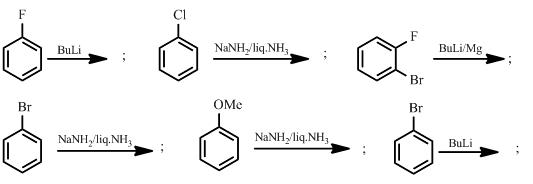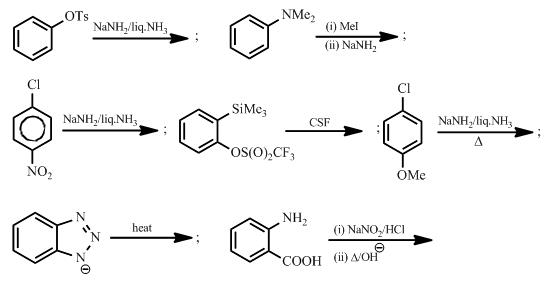Solution:
*Answer can only contain numeric values
QUESTION: 43

The total number of enantiomeric pairs possible of the given compound: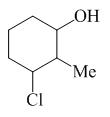Solution:
*Answer can only contain numeric values
QUESTION: 44

Number of reagents which correctly match in the reference to reaction on double bonds: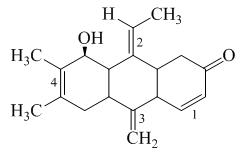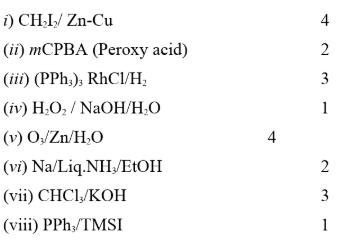Solution:
*Answer can only contain numeric values
QUESTION: 45

How many number of diene which undergoes Diels–Alder reaction with maleic anhydride is: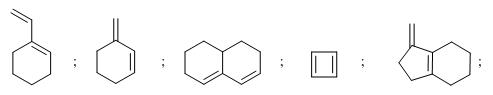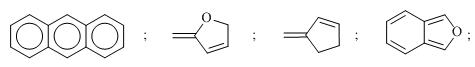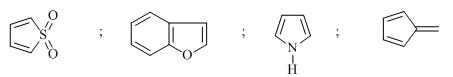Solution:
*Answer can only contain numeric values
QUESTION: 46

The  λmax of the following compound. The base value are: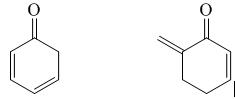Solution:
*Answer can only contain numeric values
QUESTION: 47

The number of compound which exhibit 5 signals in 1H NMR spectroscopy: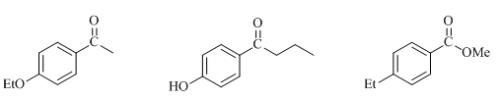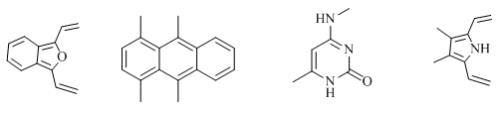Solution:
*Answer can only contain numeric values
QUESTION: 48

Number of biopolymers:

Sucrose, Galactose, Starch, Lactose, Cellulose, Amylose, Maltose, Amylopectin, DNA, RNA, Protein, Nucleotide.

Solution:
*Answer can only contain numeric values
QUESTION: 49

How many of the following are essential amino acids?

Valine, Leucine, Isolececine, Methionine, Arginine, Tryptophan, Phenylalanine, Aspargine, Glutamine, Serine, Tyrosine, Glycine, Alamin, Cysteine, Proline, Histidine:

Solution:
*Answer can only contain numeric values
QUESTION: 50

Which of the following molecules will be microwave active?

HCl; N2; CO; NO; H2; Benzene; SF6; CH3Cl; CH4; CH2Cl2

Solution:
*Answer can only contain numeric values
QUESTION: 51

How many number of compounds having higher chemical shift δ of B than A: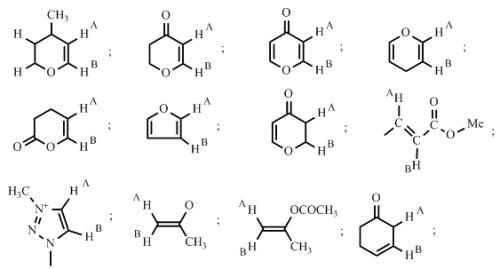Solution:
*Answer can only contain numeric values
QUESTION: 52

Among the following number of compound which reacts with active metal: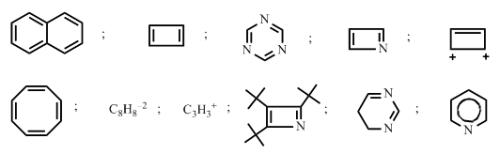Solution:
*Answer can only contain numeric values
QUESTION: 53

How many number of compound having IR – stretching frequency higher than 1720: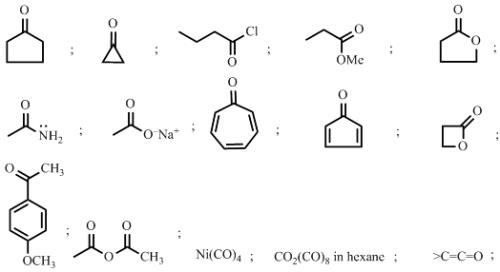Solution:
*Answer can only contain numeric values
QUESTION: 54

Find the number of RMgX used in the product: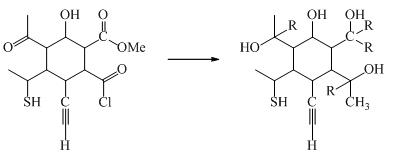Solution:
*Answer can only contain numeric values
QUESTION: 55

How many number of reaction proceed through NGP.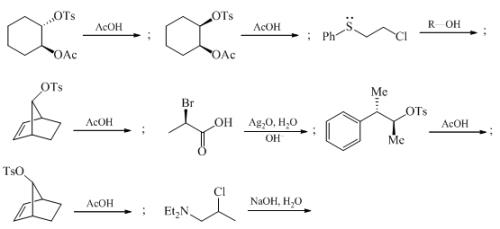Solution:
*Answer can only contain numeric values
QUESTION: 56

Among the following the number of reaction which shows radical coupling: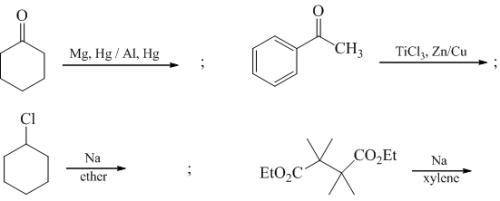Solution:
*Answer can only contain numeric values
QUESTION: 57

Among the following the number of reaction which showscarbene intermediate: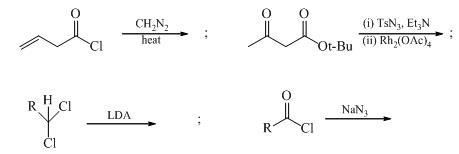Solution:
*Answer can only contain numeric values
QUESTION: 58

No of correct match of the following amino acids in reference to their isoelectric points.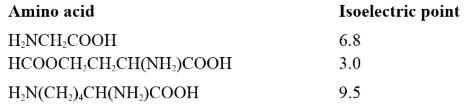Solution:
*Answer can only contain numeric values
QUESTION: 59

Number of aromatic compounds?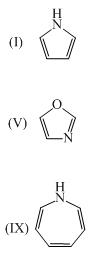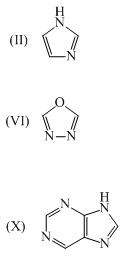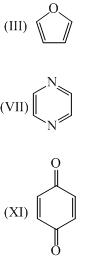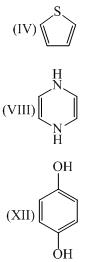Solution:
*Answer can only contain numeric values
QUESTION: 60

Total number of bending modes of vibrations (collectively) in chloroform & Acetylene would be __________?

Solution: